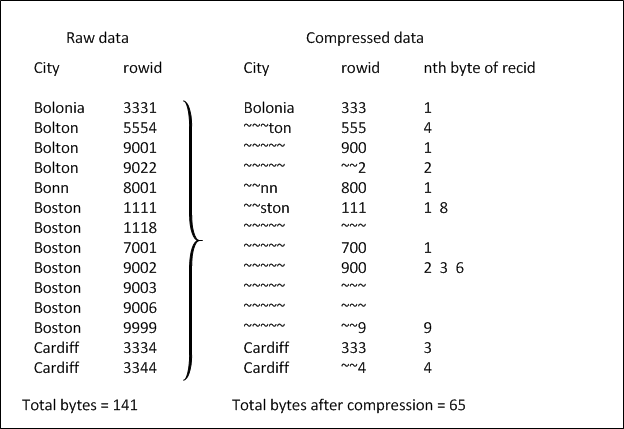Database Essentials
Database Design : Defining indexes : Calculating index size

# Calculating index size

You can estimate the approximate maximum amount of disk space occupied by an index by using this formula:
 Number of rows * (7 + number of columns in index + index column storage) * 2
For example, if you have an index on a character column with an average of 21 characters for column index storage and there are 500 rows in the table, the index size is:
 500 * (7 + 1 + 21) * 2 = 29,000 bytes
The size of an index is dependent on four things:The number of entries or rows.The number of columns in the key.The size of the column values, that is, the character value "abcdefghi" takes more space than "xyz." In addition, special characters and mulit-byte Unicode characters take even more space.The number of similar key values.
You will never reach the maximum because OpenEdge uses a data compression algorithm to reduce the amount of disk space an index uses. In fact, an index uses on average about 20% to 60% less disk space than the maximum amount you calculated using the previously described formula.
The amount of data compressed depends on the data itself. OpenEdge compresses identical leading data and collapses trailing entries into one entry. Typically non-unique indexes get better compression than unique indexes.
Note: All key values are compressed in the index, eliminating as many redundant bytes as possible.
The following figure shows how OpenEdge compresses data.
Figure 10. Data compressionThe City index is stored by city and by ROWID in ascending order. There is no compression for the very first entry "Bolonia." For subsequent entries, OpenEdge eliminates any characters that are identical to the leading characters of Bolonia. Therefore, for the second entry, "Bolton," it is not necessary to save the first three characters "Bol" since they are identical to leading characters of Bolonia. Instead, Bolton compresses to "ton." Subsequently, OpenEdge does not save redundant occurrences of Bolton. Similarly, the first two characters of "Bonn" and "Boston" ("Bo") are not saved.
For ROWIDs, OpenEdge eliminates identical leading digits. It saves the last digit of the ROWID separately and combines ROWIDs that differ only by the last digit into one entry. For example, OpenEdge saves the leading three digits of the first ROWID 333 under ROWID, and saves the last digit under nth byte. Go down the list and notice that the first occurrence of Boston has a ROWID of 1111, the second has a ROWID of 1118. Since the leading three digits (111) of the second ROWID are identical to the first one, they are not saved; only the last digit (8) appears in the index.
Because of the compression feature, OpenEdge can substantially decrease the amount of space indexes normally use. In the above figure, only 65 bytes are used to store the index that previously took up 141 bytes. That is a saving of approximately 54%. As you can see, the amount of disk space saved depends on the data itself, and you can save the most space on the non-unique indexes.